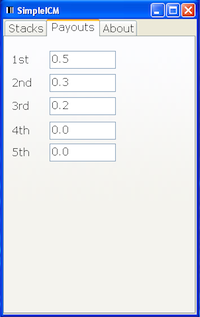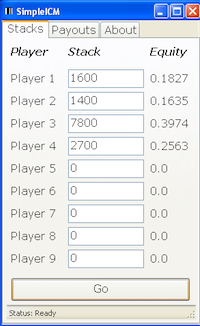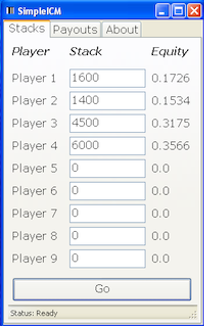SimpleICM is a basic ICM calculator. Given the payout structure and the stacks of the remaining players it calculates the tournament equity for each player. It doesn’t tell you whether to push or fold or calculate your equity against an opponents hand range. There are other tools that can do that.

But it can help your understanding of ICM to start from the basic ICM calculation. This posts shows an example of using SimpleICM to perform an equity calculation and how to use that calculation to decide what action to take.

#### Structure

For this example we’ll use a 9-player Sit-n-go with the standard 50, 30, 20 payout structure. So the top 3 players get paid with 1st getting 50% of the prize pool, second getting 30% of the prize pool and 3rd getting 20% of the prize pool.This is the default payout structure in SimpleICM so we don’t need to change anything on the payout tab.

#### Stacks

There are 4 players left and we’re in the BB with AKs. The stack sizes are:

PositionStackHandAction
CO1600Fold
BTN1400Fold
SB7500???Push
BB (Hero)3000AKs???

#### Action

The blinds are 150/300. CO and BTN both fold. SB pushes all-in. What do you do?

#### Equity

So now we get to start plugging some numbers into SimpleICM. If we fold we will be left with 2700 chips after posting the bb and SB will have 7800. We can plug these stacks into SimpleICM to get our equity if we fold.Equity of foldingEquity of calling and winning

Calling can be split into two events. If we call and lose then our equity is 0. If we call and win then we will have 6000 chips so we can plug these stacks into SimpleICM to get our equity.

So,

• If we fold our equity will be 0.2563
• If we call and lose our equity will be 0
• If we call and win our equity will be 0.3566

So for calling to be profitable here we need to win around 72% of the time ((0.2563/0.3566)*100).